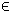## 9QPCON Problem

When solving a problem with a quadratic objective and nonlinear constraints TOMLAB automatically supplies objective derivatives (gradient and Hessian) if qpconAssign is used.

The quadratic constrained nonlinear programming problem is defined as:

 min x
f(x) =
1
2
xT F x + dT x

s/t
 xL ≤ x ≤ xU bL ≤ A x ≤ bU cL ≤ c(x) ≤ cU
(11)
where x, xL, xU, dRn, FRn × n, f(x)R, ARm1 × n, bL,bURm1 and cL,c(x),cURm2.

The following files define and solve an example problem in TOMLAB.

File: tomlab/quickguide/qpconQG.m, qpconQG_c.m, qpconQG_dc.m, qpcon_d2c.m
```  c:   Nonlinear constraint vector
d2c: The second part of the Hessian to the Lagrangian function for the nonlinear constraints.
```
The following file illustrates how to solve this QPCON problem in TOMLAB. Also view the m-files specified above for more information.

File: tomlab/quickguide/qpconQG.m

Open the file for viewing, and execute qpconQG in Matlab.
```% qpconQG is a small example problem for defining and solving quadratic
% nonlinearly constrained programming problems using the TOMLAB format.

Name  = 'QP constrained problem';
x_0   = ones(10,1);
x_L   = [];
x_U   = [];
A     = ones(8,10);
for i = 1:8
A(i,i) = 1/2;
end
b_L   = ones(8,1);
b_U   = ones(8,1);
c_L   = 4;
c_U   = 4;

% Objective f = -x'*x + sum(x), assign as quadratic/linear matrix/vector

F     = -2*speye(10);
d     = ones(10,1);

Prob = qpconAssign(F, d, x_L, x_U, Name, x_0, A, b_L, b_U,...
'qpconQG_c', 'qpconQG_dc', 'qpconQG_d2c', [], c_L, c_U);

% Run SNOPT as a local solver
Result = tomRun('snopt', Prob, 1);
% Result2 = tomRun('minos', Prob, 1);
% Result3 = tomRun('npsol', Prob, 1);
% Result4 = tomRun('knitro', Prob, 1);```